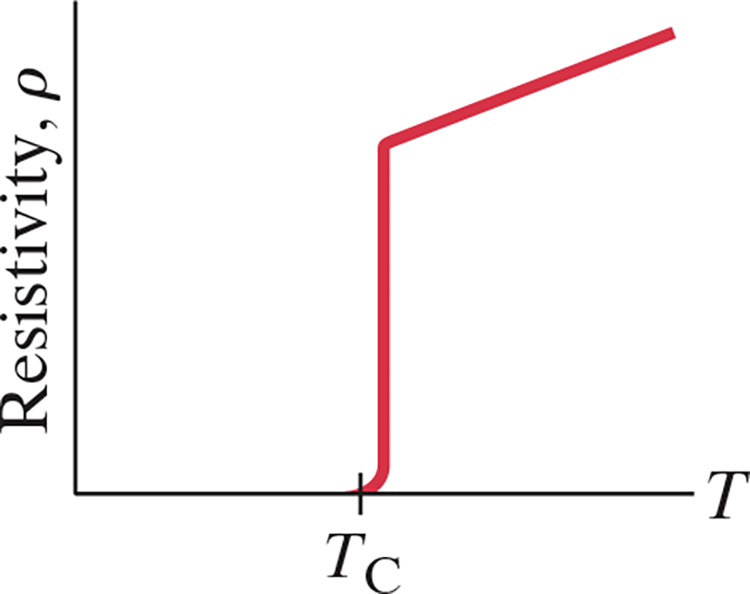# Notes can be found as interactive webpage at

25: Electric Current and Resistance

# 25.1 The Electric Battery #

• Batteries produce electricity by transforming chemical energy into electric energy
• Simple battery (cells) contain two plates or rods of dissimilar metals called electrodes
• The portion of rods outside of the solution are called the terminals
• Anode: The positive electrode
• Cathode: The negative electrode
• These electrodes are emersed in the electrolyte: a solution such as a dilute acid

Chemical Process:

1. The acid dissolve the zinc electrode, causing zinc atoms to leave two electrons behind on the electrode and enters the solution as a positive ion. The zinc electrode thus acquires a negative charge.
2. Then the electrolyte becomes positively charged and can pull electrons off the carbon electrode. Thus the carbon electrode becomes positively charged.
3. Because there is an opposite charge on the two electrodes, there is a potential difference between the two terminals.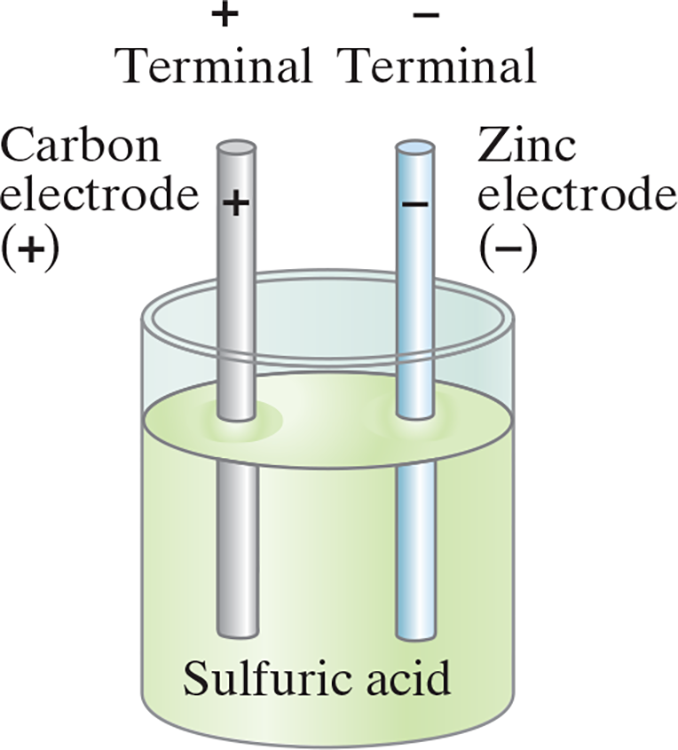• When a battery isn’t connected, only a small amount of zinc is dissolved
• The zinc electrode becomes increasingly negative
• Thus, any new positive zinc ions produced are attracted back to the electrode
• That is, if a charge is allowed to flow then the zinc can dissolve
• The voltage depends ot the electrodes’ material and their relative ability to give up electrons

# 25.2 Electric Current #

• When a circuit is formed, charge can move (flow) through the wires from one terminal to the other
• Any flow of charge is called an electric current
• Flow can only occur on a continuos conducting path (a complete circuit)
• If there’s any break, our circuit is called an open circuit and no current flows
• The symbol for battery is the following: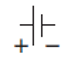Conventional current from .$+$ to .$-$ is equivalent to a negative electron flow from .$-$ to .$+$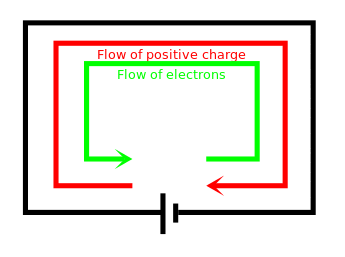• Current in a wire is defined as the net amount of charge that passes through the wire’s full cross section at any point in time: $$\bar I = \frac{\Delta Q}{\Delta t} \Longrightarrow I = \frac{dQ}{dt}$$
• Current is measured in coulombs per second; ampere (amp): .$\text{1 A = 1 C/s}$

# 25.3 Ohm’s Law: Resistance and Resistors #

• For a current to exist, there must be a potential difference (e.g. between the terminals of a battery)
• That is, the current is proportional to the potential difference: $$I \propto V$$
• E.x., a wire connected to a .$6V$ battery results in a current twice that of a .$3V$ battery
• The current depends on the resistance that the wires offers
• The electron flow is impeded partly due to the atoms in the wire
• .$R$ is this proportionality factor between voltage and current
• Thus, we get Ohm’s Law: $$V = IR$$
• Ohm’s law only works for when .$R$ is a constant, i.e a metal conductor
• In reality, .$R$ isn’t constant if temperature changes much
• Materials that follow Ohm’s law are labeled as “ohmic”
• Resistance has the units/notation .$\text{1$\Omega$= 1 V/A}$
• Resistors are used to limit/control the current in a circuit
• toolbox.mehvix.com/resistor
• As a current passes through a resistor, the charge/current stays the same but the electric potential decreases

Clarifications of Behavior

• Current’s magnitude depends on that device’s resistance
• Can be though of as the “response” to the voltage: increases if voltage increases or resistance decreases
• Current is constant – it’s energy so it cannot be destroyed by components and it’s not created by a battery
• Resistance is a property of the device/wire
• Voltage is external to the wire of device – it’s applied across the two ends of the wire
• Batteries maintain a constant potential difference – act as a source of voltage

# 25.4 Resistivity #

• Resistivity has experimentally been found as $$R = \rho \frac{l}{A}$$
• .$\rho$ is the resistivity (constant of proportionality) and depends on the material
• Has units .$\Omega \cdot \text{m = V/A$\cdot $m}$
• .$l$ is the wire length
• .$A$ is the cross-section area
• The reciprocal of resistivity is conductivity: .$\sigma = \rho^{-1}$

## Temperature #

• Resistivity varies (generally increasing) with temperature $$\rho_T = \rho_0 = \bigg[ 1+ \alpha (T-T_0)\bigg]$$
• .$\rho_0$ is the resistivity at some reference temperature .$T_0$ (i.e .$0^\circ \text{ C}$)
• .$\rho_T$ is the new resistivity at the current (higher) temperature .$T$
• .$\alpha$ is the temperature coefficient of resistivity that depends on material
• Note that the temperature coefficient for semiconductors can be negative.
• At higher temperatures, some of the electrons that are normally not free in a semiconductor can become free and contribute to the current.
• Thus, the resistance of a semiconductor can decrease with an increase in temperature.

# 25.5 Electric Power #

• Electric energy is transformed into thermal energy (and light) in stove burners, toasters, etc.
• The current creates collisions between the moving electrons and the atoms in the wire
• That is, the KE from the wire’s atoms increases meaning the temperature increases too $$P = \frac{dU}{dt} = \frac{dq}{dt}\cdot V$$
• This is because energy is transformed when a tiny charge .$dq$ moves through a potential difference .$V$ is .$dU = V\ dq$
• The charge that flows per second, .$dq/dt$, is the electric current .$I$: $$P = IV = I^2 R = \frac{V^2}{R}$$
• The SI unit for power is the watt: .$\text{1 W = 1 J/s}$
• We get the last two equations by plugging in .$V = IR$

# 25.7 Alternating Current #

• When a battery is connected to a circuit, the current moves steadily in one direction (DC: Direct Current)
• Electric generators at power plants produce AC: alternating current
• Reverses direction many times per second and is commonly sinusoidal $$V = V_0 \sin(2\pi ft) = V_0 \sin(\omega t)$$
• .$\omega$ = .$2\pi f$
• .$f$ is the frequency: number of complete oscillations per second
• Commonly .$\text{60 Hz}$ in NA
• Potential .$V$ oscillates between .$\pm V_0$, the peak voltage
• Current equation still works: $$I = \frac{V}{R} = \frac{V_0}{R}\sin\omega t = I_0 \sin\omega t$$
• .$I_0 = V_0/R$ is the peak current
• Avg current is 0; it’s positive and negative for an equal amount of time
• Doesn’t mean that no heat is created or no power is needed
• Electrons are still moving though!
• Power is also consistent $$P = I^2R = I_0^2 R \sin\omega t = \frac{V_0^2}{R} \sin\omega t$$
• Power is always positive because current is squared
• Since the .$\sin\dots$ oscillates between 1 and 0, the average power is $$\overline P = \frac{1}{2}I_0^2R = \frac{1}{2} \frac{V_0^2}{R}$$
• This can also be calculated by using the RMS values for .$I$ and .$V$ $$I_\text{rms} = \sqrt{\overline I^2} = \frac{I_0}{\sqrt{2}} \approx 0.707 I_0$$ $$V_\text{rms} = \sqrt{\overline V^2} = \frac{V_0}{\sqrt{2}} \approx 0.707 V_0$$ $$\dots \Longrightarrow \overline P = I_\text{rms} V_\text{rms} = I_\text{rms}^2 R = \frac{V_\text{rms}^2}{R}$$
• Fun fact: we can use the rms of a value to find the peak of it, e.x. $$V_0 = \sqrt{2} V_\text{rms}$$
• Keep in mind that this is the average power. Instantaneous power varies from .$0$ to .$2\overline P$

# 25.8 Microscopic View of Current #

• We’ve seen that electric current can be carried by negatively charged electrons in metal wires, and that in liquid solutions current can also be carried by positive and/or negatively charged ions
• When a potential difference is applied to the two ends of a wire, the direction of the electric field .$\vec E$ is parallel to the walls of the wire
• This field within the conducting wire does not contradict our earlier result that .$\vec E = 0$ inside a conductor in the electrostatic case, as we are no longer dealing with the static case.
• That is, charges are free to move in a conductor, and hence can move under the action of the electric field.
• If all the charges are at rest, then .$\vec E = 0$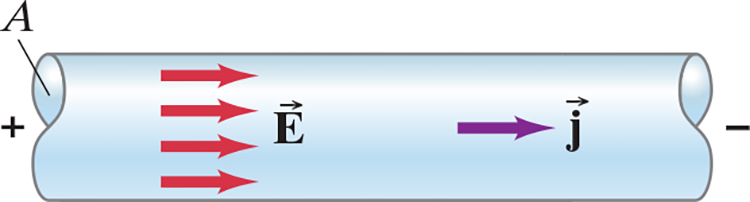## Current Density #

• Current density, .$\vec j$, is the current per area $$j = \frac{I}{A} \Longrightarrow I = \int \vec j \cdot d \vec A$$
• .$I$ is the current through the whole surface
• .$d\vec A$ is an element of surface area over which the integration is taken
• Direction of the density is the same direction as .$\vec E$ – the direction that a positive charge would move

## Drift Speed #

• Inside a wire, we can imagine the free electrons as moving about randomly at high speeds, bouncing off the metal atoms of the wire
• Somewhat like the molecules of a gas
• When an electric field exists in the wire the electrons feel a force and initially begin to accelerate but they soon reach a more or less steady average speed, known as their drift speed .$v_d$
• Collisions with atoms in the wire keep them from accelerating further
• The drift speed is normally very much smaller than the electrons’ average random speed inside the metal wire

Black zagged line represents the motion of an electron in a metal wire due to an electric field. The field .$\vec E$ gives electrons in random motion a net drift velocity .$\vec v_d$. Its direction (the net charge flow) is in the opposite direction of .$\vec E$ because electrons have a negative charge and .$\vec F = q \vec E$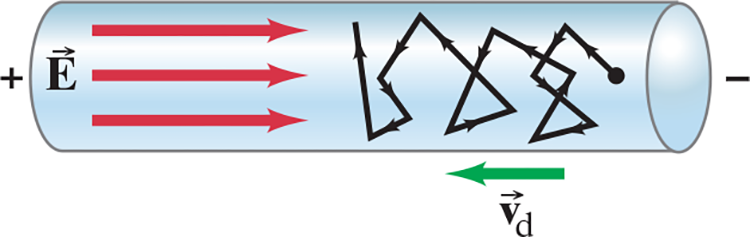• We can relate drift speed with the macroscopic view:
• In some time, the electrons travel (the average) distance .$l = v_d \Delta t$
• In that same time, electrons in volume .$V = Al = A v_d \Delta t$ pass through area .$A$ of the wire
• If there are .$n$ free electrons each of charge .$-e$ per unit volume, then the total electrons is .$N = nV$
• Thus, the charge is $$\Delta Q = \text{(number of charges, N)\times(charge per particle, -e)}$$ $$\dots = (nV)(-e) = -(nAv_d\Delta T)(e)$$
• We can then easily find the current (density): $$I = \frac{\Delta Q}{\Delta t} = -neAv_d$$ $$j = \frac{I}{A} = -nev_d$$
• Notice that the negative sign indicates that the direction of (positive) current flow is opposite to the drift speed of electrons.

## Field inside a Wire #

• Voltage can be written in terms of microscopic values (in addition to the macro: .$V = IR$)
• Recall that resistance is related to density by .$R = \rho \frac{l}{A}$
• We can then write .$V$, .$I$ and .$j$ as

$$V = El = IR = (jA)\bigg(\rho \frac{l}{A}\bigg) = j \rho l$$

$$I = jA$$

$$j = \frac{1}{\rho}E = \sigma E$$

• .$\sigma$ is the conductivity of the wire
• .$\rho, \sigma$ do not vary with .$V$ and thus neither .$E$
• We can then write the microscopic statement of Ohm’s Law: $$\vec j = \sigma \vec E = \frac{\vec E}{\rho}$$

# 25.9 Superconductivity #

• At very low temperatures, the resistivity of certain metals and certain compounds or alloys becomes zero
• Materials in such a state are said to be superconducting
• In general, superconductors become superconducting only below a certain transition (critical) temperature, .$T_C$